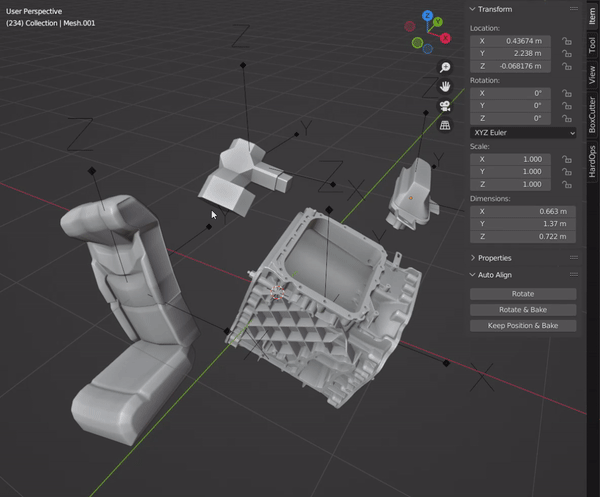# Auto Align | Automatically re-aligns wrong axis objects

This is a simple add-on that automatically re-aligns wrong axis objects.## Usage

There are three options available in the `3D Viewport > Sidebar > Item > Auto Align`.

• `Rotate` : Rotate the selected object to match the world axis.
• `Rotate & Bake` : Same as `Rotate`, but rotation is applied.
• `Keep Position & Bake` : Keep the object fixed and only change the local axis.

Also there is a `symmetry` option. This option can be used to align symmetrical objects, even though there are not many orthogonal faces to the axis. It’s a bit slower, but effective when aligning organic objects, such as people.This is similar to World Align add-on, but with automatic detection of appropriate axis (and also free!).

4 Likes

## Algorithm

The proper orientation of the object is calculated as follows:

1. RANSAC (Random sample consensus)

• Randomly select two orthogonal faces to create a candidate orientation.
• Find the faces that corresponding to the axis within a specific threshold (<5 deg).
• Criterion is to maximize the sum of the areas of the face.
2. Correction

• Fine-tune the candidate orientation.
• Find the weighted median of the points on each axis and rotate the orientation.
• Repeat until convergence.

• To avoid continuous flips and maintain consistency while repeating the same operation.
• Determine XYZ axis to have the least difference from the initial mesh orientation.

For each object, only up to 10000 faces are considered in the process. This is due to performance reason. If there are more than 10000 faces, larger faces would be considered first.

3 Likes

Implemented the algorithm that aligning symmetrical object.### Symmetric Plane Detection Algorithm

When the `symmetry` option is on, the symmetry plane must be derived before RANSAC.

1. Vertex pair matching
• Randomly extract vertex pairs that satisfy symmetry conditions.
• Vertex normals must be consistent with their relative positions.
2. Plane voting
• 4 parameters of fitting plane is calculated from each pair.
• From 4D voting space, choose plane parameters that receive the most votes.
3. Correction
• From the planes within a certain distance, calculate the median of each parameter.

### Note

• When using `symmetry` option, the model doesn’t need to be completely symmetrical because the algorithm considers the outlier.
• The symmetry plane detection algorithm is far from perfect. If the object is complex (>105 vertices?), symmetry detection will fail with a high probability. Also, if a single object has multiple planes of symmetry, the alignment can be done in an unintended manner.
3 Likes